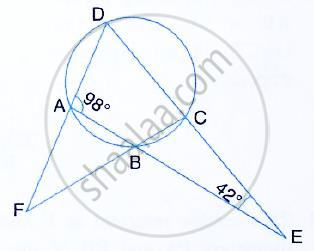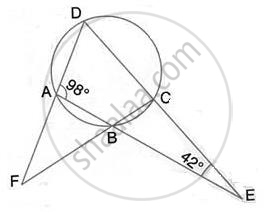Share

# The Sides Ab and Dc of a Cyclic Quadrilateral Abcd Are Produced to Meet at E; the Sides Da and Cb Are Produced to Meet at F. If ∠Bec = 42° and ∠Bad = 98°; Calculate : (I) ∠Afb (Ii) ∠Adc - Mathematics

Course

#### Question

The sides AB and DC of a cyclic quadrilateral ABCD are produced to meet at E; the sides DA and CB are produced to meet at F. If ∠BEC = 42° and ∠BAD = 98°; Calculate :#### SolutionBy angle sum property of ∆ADE,
∠ADC = 180° - 98° - 42° = 40°
(pair of opposite angles in a cyclic quadrilateral are supplementary)
∴ ∠ABC = 180° - 40° = 140°
Also, ∠BAF = 180° - ∠BAD = 180° - 98° = 82°
∴ ∠ABC = ∠AFB + ∠BAF
(Exterior angle of a ∆ is equal to the sum of pair of interior opposite angles)
⇒ ∠AFB =140° - 82° = 58°
Thus, ∠AFB = 58°  and ∠ADC = 40°

Is there an error in this question or solution?